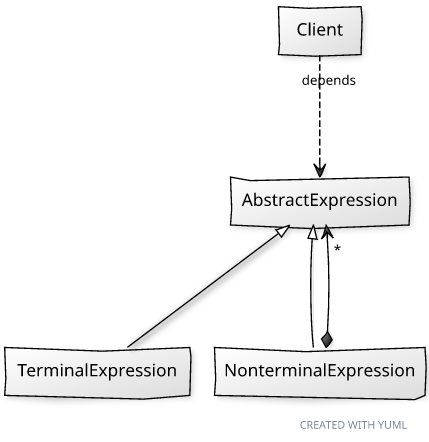## Thursday, February 18, 2010

### Practical Php Patterns: Interpreter

This post is part of the Practical Php Pattern series.

The behavioral pattern of today is the Interpreter pattern, which consists in a representation of a grammar with a Composite class hierarchy, where rules are mapped to classes. An expression that follows the grammar can thus be translated to an abstract syntax tree, which is nothing else than an object graph, instance of the Composite pattern.
The abstract adjective for the tree was chosen because it is in fact the most abstract representation of an expression, ignoring the concrete ones it may have as a string or as an other data structure (e.g. in php "A" and "\x41" are different concrete representations of the same abstract literal.) The resulting decoupling of logic rules from presentation purposes is a great simplification of the interpretation process.
Interpreter is not a very common pattern, but for simple grammars it makes adding new rules as easy as adding a class. It does not address the transformation from the concrete representation, which is done by other services, to the abstract syntax tree.Terminology
The point of this pattern is leveraging the Composite hierarchy for a simple implementation of the AbstractExpression methods (Interpreter operations.)
The arguments of the Interpreter operations are usually collectively called context. Given a method signature, they are usually values to substitute in calculations, parameters for the operations, or they may not exist at all for certain operations.
Similarly, the Leaf and Container participants of the Composite patterns assume different names when an Interpreter is involved. These names reflect their played roles: terminal and nonterminal expression.

Participants:
• Client: uses the Interpret operations.
• AbstractExpression: abstraction over an expression tree.
• NonTerminalExpression: expression which recursively contains other AbstractExpression instances.
• TerminalExpression: expression which cannot be simplified further in more than one object.
The GoF bookhas an extended example of this pattern; I am going to revisit it by using mathematical expressions instead of boolean ones.
Thus the example addresses the representation of a mathematical expression, and the separation of its evaluate() operation concerns in the different ConcreteExpression classes.
```<?php
/**
* AbstractExpression. All implementations of this interface
* are ConcreteExpressions.
*/
interface MathExpression
{
/**
* Calculates the value assumed by the expression.
* Note that \$values is passed to all expression but it
* is used by Variable only. This is required to abstract
* away the tree structure.
*/
public function evaluate(array \$values);
}

/**
* A terminal expression which is a literal value.
*/
class Literal implements MathExpression
{
private \$_value;

public function __construct(\$value)
{
\$this->_value = \$value;
}

public function evaluate(array \$values)
{
return \$this->_value;
}
}

/**
* A terminal expression which represents a variable.
*/
class Variable implements MathExpression
{
private \$_letter;

public function __construct(\$letter)
{
\$this->_letter = \$letter;
}

public function evaluate(array \$values)
{
return \$values[\$this->_letter];
}
}

/**
* Nonterminal expression.
*/
class Sum implements MathExpression
{
private \$_a;
private \$_b;

public function __construct(MathExpression \$a, MathExpression \$b)
{
\$this->_a = \$a;
\$this->_b = \$b;
}

public function evaluate(array \$values)
{
return \$this->_a->evaluate(\$values) + \$this->_b->evaluate(\$values);
}
}

/**
* Nonterminal expression.
*/
class Product implements MathExpression
{
private \$_a;
private \$_b;

public function __construct(MathExpression \$a, MathExpression \$b)
{
\$this->_a = \$a;
\$this->_b = \$b;
}

public function evaluate(array \$values)
{
return \$this->_a->evaluate(\$values) * \$this->_b->evaluate(\$values);
}
}

// 10(a + 3)
\$expression = new Product(new Literal(10), new Sum(new Variable('a'), new Literal(3)));
echo \$expression->evaluate(array('a' => 4)), "\n";
// adding new rules to the grammar is easy:
// e.g. Power, Subtraction...
// thanks to the Composite, manipulation is even simpler:
// we could add substitute(\$letter, MathExpression \$expr)
// to the interface...```

#### 1 comment:

PHP Developer India said...

All are generally useful, with the only exception perhaps being the Interpreter pattern.